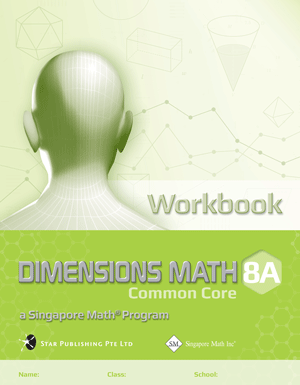# Singapore Math Dimensions Math Workbook 8A

Workbooks are the essential supplements to textbooks as they give students the opportunity to practice applying new concepts. Workbook exercises polish analytical skills and help students develop a stronger foundation in math through repetition and variation.

Features & Components:

• Basic Practice: Simple questions that drill comprehension of concepts.
• Further Practice: More difficult questions that involve direct application.
• Challenging Practice: Questions that require synthesis.
• Enrichment: Questions that demand higher order thinking, analysis, and reasoning.

The answer key at the back of the book provides answers only. Worked Solutions (sold separately) contain fully worked solutions.

Note: Two workbooks (A and B) correspond to the two halves of the school year. Soft cover.

Dimensions Math® Workbook 8A

Chapter 1: Exponents and Scientific Notation

1.1 Positive Exponents and the Laws of Exponents
1.2 Zero and Negative Integer Exponents
1.3 Fractional Exponents

A. nth root of a Positive Number
B. Fractional Exponents

1.4 Comparing Exponents
1.5 Scientific Notation

A. Representation of Very Small or Very Large Numbers
B. Computation

1.6 Rounding Numbers to a Specified Number of Significant Figures
1.7 Estimations and Accuracy of Calculators

A. Estimations
B. Estimation and Irrational Numbers
C. Accuracy in Calculators

In a Nutshell
Review Exercise 1

Chapter 2: Linear Equations in Two Variables

2.1 Linear Equations in Two Variables

A. Review of Linear Equations in One Variable
B. Meaning of Linear Equations in Two Variables

2.2 Solving Simultaneous Linear Equations in Two Variables using the Graphical Method
2.3 Solving Simultaneous Linear Equations in Two Variables using the Substitution Method
2.4 Solving Simultaneous Linear Equations in Two Variables using the Elimination Method
2.5 Solving Problems using Simultaneous Equations

In A Nutshell
Review Exercise 2

Chapter 3: Expansion and Factorization of Algebraic Expressions

3.1 Expansion of the Products of Algebraic Expressions

A. Product of Single Terms
B. Product of Two Expressions

3.2 Special Products More Properties of Inequalities
3.3 Factorization by using Special Products of Algebraic Expressions

In A Nutshell
Review Exercise 3

Chapter 4: Quadratic Factorization and Equations

4.1 Factorization of ax2 + bx +c

A. x2 + bx + c
B. ax2 + bx + c

4.2 Solving Quadratic Equations by Factorization
4.3 Problem Solving Involving Quadratic Equations

In A Nutshell
Review Exercise 4

Chapter 5: Simple Algebraic Fractions

5.1 Simplifying Simple Algebraic Fractions
5.2 Multiplication and Division of Algebraic Fractions
5.3 Addition and Subtraction of Algebraic Fractions
5.4 Fractional Equations

A. Solving Equations Involving Algebraic Fractions
B. Problem Solving Involving Fractional Equations

A. Finding the Value of an Unknown Quantity in a Formula
B. Changing the Subject of a Formula

In A Nutshell
Review Exercise 5

Chapter 6: Congruence and Reflections

6.1 Congruence and Reflections

A. Congruent Figures
B. Reflections

6.2 Translations, Rotations and Combining Transformations

A. Translations
B. Rotations
C. Combining Transformations

6.3 Similarity
6.4 Enlargements and Similarity

A. Scale Factors
B. Enlargements

In A Nutshell
Review Exercise 6

Chapter 7: Parallel Lines and Angles in Triangles and Polygons

7.1 Angles formed by Parallel Lines and Transversals

A. Angle Properties
B. Parallel Lines and Transversals

7.2 Interior and Exterior Angles of a Triangle

A. Angle Sum of a Triangle
B. Exterior Angle of a Triangle

7.3 Angles Properties of Special Quadrilaterals
7.4 Polygons

A. Types of Polygons
B. Sum of Interior Angels of a Polygons
C. Sum of Exterior Angels of a Polygons

In A Nutshell
Review Exercise 7